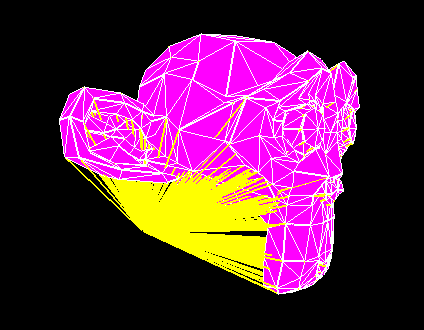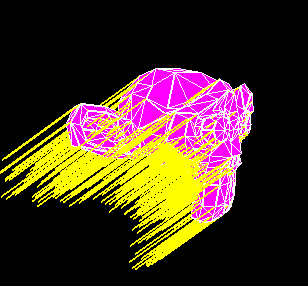# Fail to visualize normals...

Okay, so I’m trying to create a vertex buffer that will allow me to see the normals of my mesh. Problem is, while it does create the lines, they all seem to converge at a single point, and on some meshes they can’t be seen at all (though this is likely because they’re inside the mesh - I’m sure if I turned off z buffering, I’d see them). This is what it looks like:The yellow lines should be connecting each vertex to it’s normal.

Here’s the part of my code that creates said buffer:

``````// Create a vert buffer for normal visualization
size = activeHeader.numVerts * sizeof(vert_P) * 2;

if (activeNormVis)
free(activeNormVis);

vert_P* activeNormVis = (vert_P*)malloc(size);
for (i = 0; i < activeHeader.numVerts; i++)
{
activeNormVis[i*2].x = activeVertData[i].x;
activeNormVis[i*2].y = activeVertData[i].y;
activeNormVis[i*2].z = activeVertData[i].z;

activeNormVis[(i*2)+1].x = activeVertData[i].nx;
activeNormVis[(i*2)+1].y = activeVertData[i].ny;
activeNormVis[(i*2)+1].z = activeVertData[i].nz;
}

glGenBuffers(1, &nbo);
glBindBuffer(GL_ARRAY_BUFFER, nbo);
glBufferData(GL_ARRAY_BUFFER, size, activeNormVis, GL_STATIC_DRAW);
``````

and here’s the part that displays it:

``````glBindBuffer(GL_ARRAY_BUFFER, nbo);
glVertexAttribPointer(0, 3, GL_FLOAT, GL_FALSE, 12, NULL);
glUniform4f(uniformColor, 1, 1, 0, 1);

``````

What am I doing wrong?

I guess they don’t converge on a single point, but on several points that lie on a unit sphere around the origin…

You ask OpenGL to draw lines from the vertex coordinates to the coordinates of the normal vector, to draw lines in the normal direction you should set the second vertex to position + scale * normal (the scale defines the length of the lines).

They don’t converge at the origin, but rather off to the side (if I had to guess, at about (-0.3, -0.1, -0.2) ), also I don’t use any scaling at all, only rotation and translation, and the point is transformed along with the rest of the mesh.

Shouldn’t that be something like :
activeNormVis[(i*2)+1].x = activeVertData[i].x + activeVertData[i].nx;

activeNormVis[i*2].x = activeVertData[i].x;
activeNormVis[(i*2)+1].x = activeVertData[i].nx;

This is not good as long as nx is the x normal component.

Progress. I tried “activeNormVis[(i*2)+1].x = activeVertData[i].x + activeVertData[i].nx;” as per ZbuffeR’s suggestion, which seems to kinda fix it except now all my normals point in the same direction:Unfortunately, because I’m loading from the .3ds format, I need to calculate my own normals, which could be the cause of this. Here’s the code I use to calculate normals:

``````	  //////////////////////////////
// Calculate vertex normals //
//////////////////////////////
for (i = 0; i < numFaces; i++)
{
Normal tempNorm;

// Calculate the surface normal
Normal U;
U.x = (verts[faces[i].b].x - verts[faces[i].a].x);
U.y = (verts[faces[i].b].y - verts[faces[i].a].y);
U.z = (verts[faces[i].b].z - verts[faces[i].a].z);

Normal V;
V.x = (verts[faces[i].c].x - verts[faces[i].a].x);
V.y = (verts[faces[i].c].y - verts[faces[i].a].y);
V.z = (verts[faces[i].c].z - verts[faces[i].a].z);

tempNorm.x = (U.y * V.z) - (U.z * V.y);
tempNorm.y = (U.z * V.x) - (U.x * V.z);
tempNorm.z = (U.x * V.y) - (U.y * V.x);

// Add the surface normal to every connected vertex
verts[faces[i].a].nx += tempNorm.x;
verts[faces[i].a].ny += tempNorm.y;
verts[faces[i].a].nz += tempNorm.z;

verts[faces[i].b].nx += tempNorm.x;
verts[faces[i].b].ny += tempNorm.y;
verts[faces[i].b].nz += tempNorm.z;

verts[faces[i].b].nx += tempNorm.x;
verts[faces[i].b].ny += tempNorm.y;
verts[faces[i].b].nz += tempNorm.z;
}

for (i = 0; i < numVerts; i++)
{
// Normalize vertex normals
float length = sqrt((verts[i].nx*verts[i].nx) + (verts[i].ny*verts[i].ny) + (verts[i].nz*verts[i].nz));

verts[i].nx /= length;
verts[i].ny /= length;
verts[i].nz /= length;
}
``````

PS. Yes, the scale in that picture is correct, the mesh is just really small.

The “Add the surface normal to every connected vertex” is not good. They can’t be the connected vertices, but are only the 3 vertices of your faces (the ones you used for calculating your cross product). These vertices can’t be the same.

Well, yes, that’s what I meant by it. It adds the value of the surface normal to each vertex that makes up that face, the same vertices which were used in the cross product to get the surface normal, which is then normalized afterward.

This is wrong. You must add normals for each face where the same vertex is shared.

But I’m trying to calculate vertex normals, not surface normals. You’re saying I’m doing it completely backwards…?

a) You have to initialize the .nx, .ny and .nz components to zero before you calculate your normals, maybe you just didn’t post that part.

b) You add the normal vector twice to point b, the last block should be

``````
verts[faces[i].c].nx += tempNorm.x;
verts[faces[i].c].ny += tempNorm.y;
verts[faces[i].c].nz += tempNorm.z;

``````

c) You calculate the sum of the tempNorm vectors for every surface. The length of this vector is proportional to the size of the surface, so smaller surfaces will have less influence on the resulting normal. This may be what you want, but often the surface normals are normalized before they are added to compute an average normal vector.

``````